# Estimating a proportion from a sample

Here, we show how to get a confidence interval for the proportion of the mean obtained from a sample.

Let us generate 100 random flips of a biased coin (p=1/3)

n <- 100
a <- rbinom(n, size=1, prob=1/3)

To obtain descriptive statistics, one can use the functions table or prop.table:

table(a)
## a
##  0  1
## 61 39
prop.table(table(a))
## a
##    0    1
## 0.61 0.39
mean(a)
##  0.39

Note the existence of two other functions that also tabulate the content of discrete variable(s) — ftable and xtabs — and offer more flexibility to display the output. The latter, xtab is particularily powerful for crosstabulating several variables.

You can plot the distributions of values using stick plots or barplots.

plot(table(a))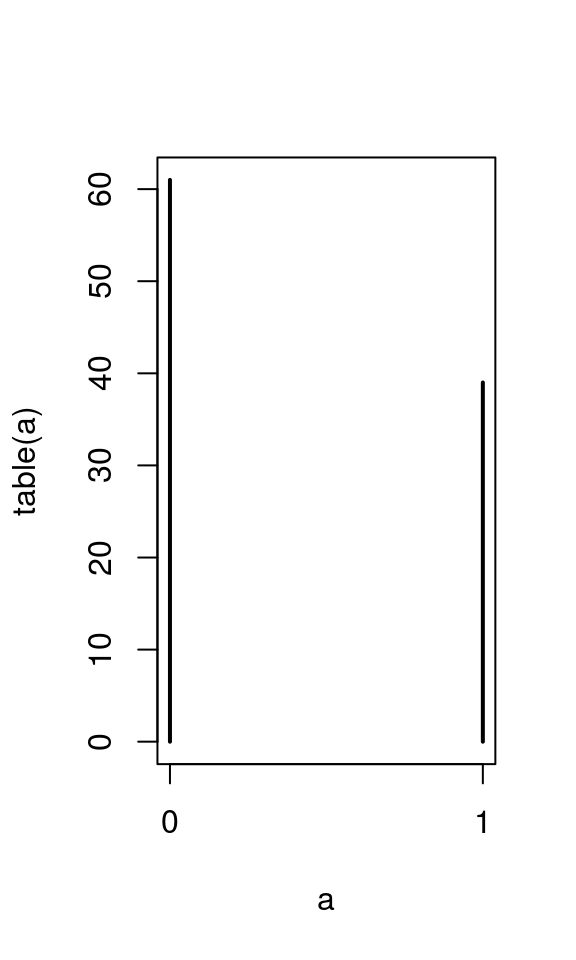barplot(table(a))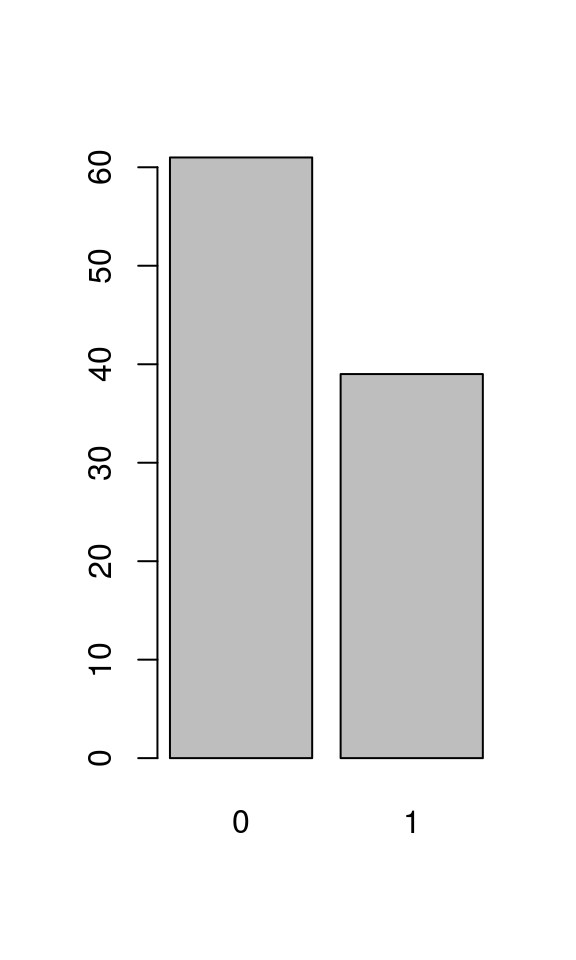The relevant function to test against the null hypothesis of p=0.5 and to obtain a confidence interval is prop.test:

prop.test(table(a))
##
##  1-sample proportions test with continuity correction
##
## data:  table(a), null probability 0.5
## X-squared = 4.41, df = 1, p-value = 0.03573
## alternative hypothesis: true p is not equal to 0.5
## 95 percent confidence interval:
##  0.5070114 0.7044326
## sample estimates:
##    p
## 0.61
prop.test(table(a))$conf.int ##  0.5070114 0.7044326 ## attr(,"conf.level") ##  0.95 ## Comparing two empirical proportions Suppose we have two binomial samples. To test wehterh the underlyinh probability is the same, we enter the counts in a 2x2 matrix where each column gives the counts of successes and failures and use prop.test again: twoprop <- matrix(c(105, 366, 45, 67), nrow=2) prop.test(twoprop) ## ## 2-sample test for equality of proportions with continuity ## correction ## ## data: twoprop ## X-squared = 14.226, df = 1, p-value = 0.0001621 ## alternative hypothesis: two.sided ## 95 percent confidence interval: ## -0.23061397 -0.05991721 ## sample estimates: ## prop 1 prop 2 ## 0.7000000 0.8452656 See also fisher.test. # Estimating the mean of a population from a sample Let us now consider the case of a continuous variable. n <- 100 a <- rnorm(n, mean=100, sd=15) ## Exploratory graphics There are several ways to graphically inspect a continous variable. Which one is approriate depends on the number of data points and their distribution. par(las=1) stripchart(a, method='jitter', vertical=TRUE) abline(h=mean(a), lty=2)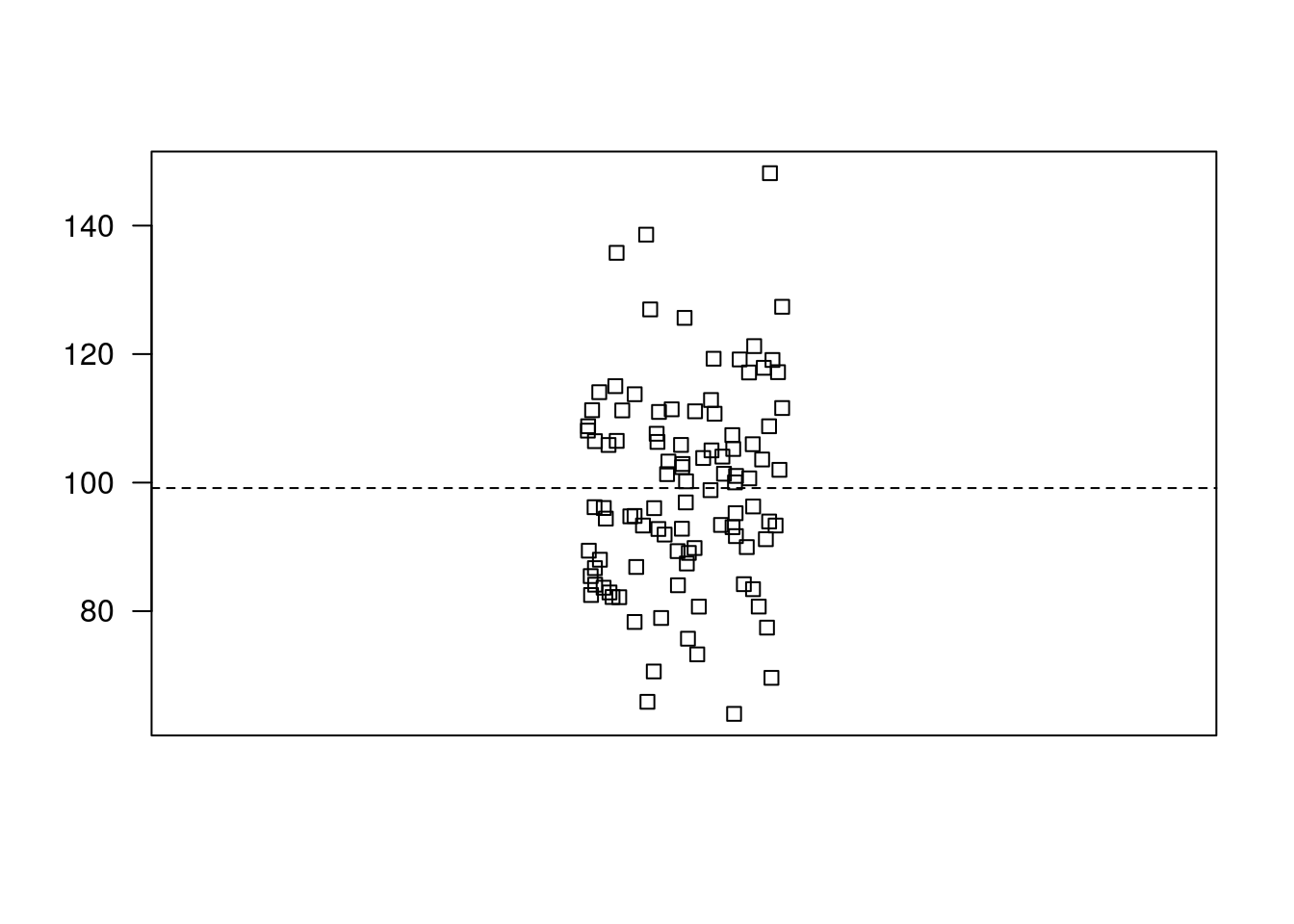If the sample is small, I recommend using a dotchart: dotchart(a[1:20])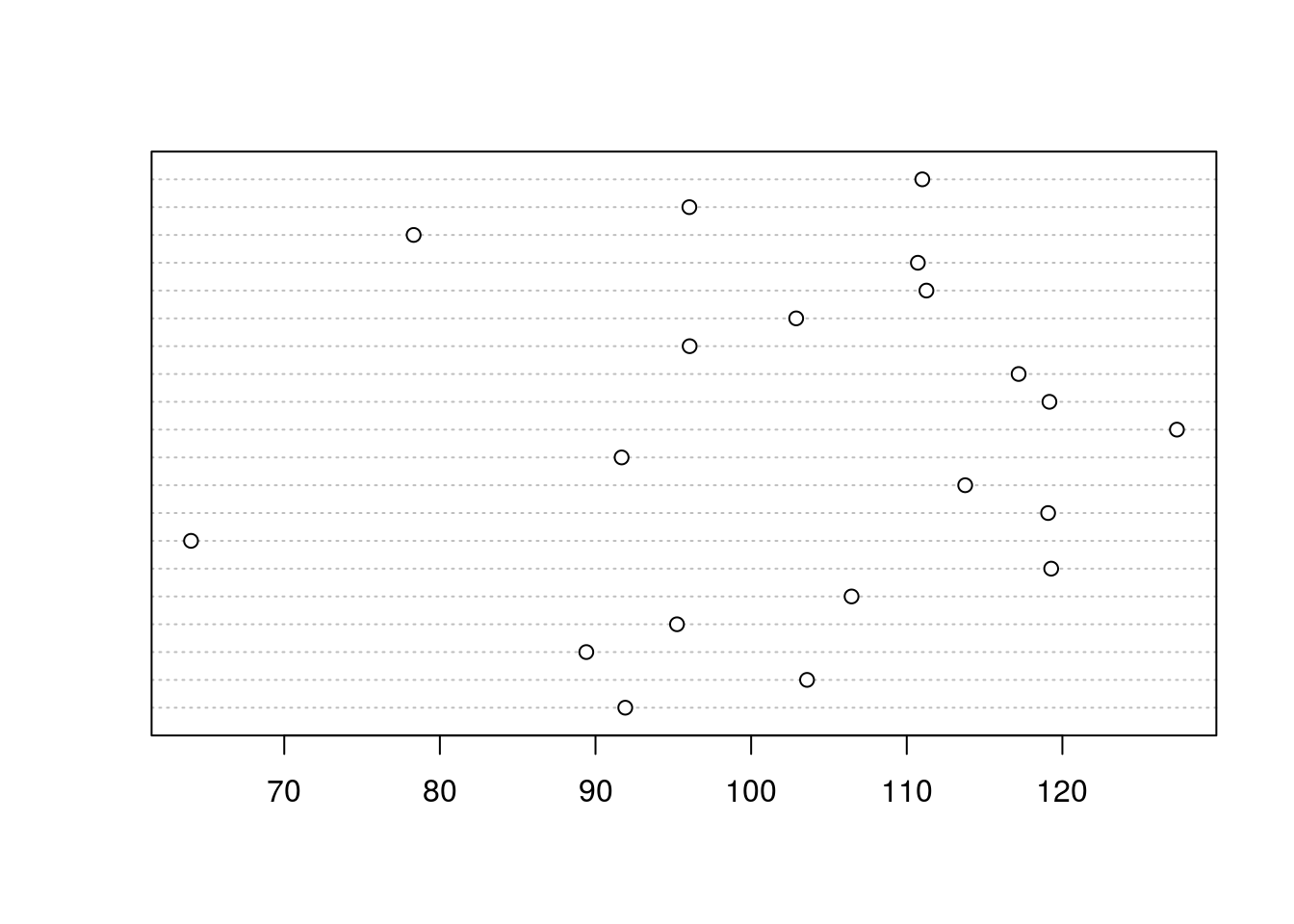If the sample is large enough, histograms or density plots are a good idea: hist(a) rug(a)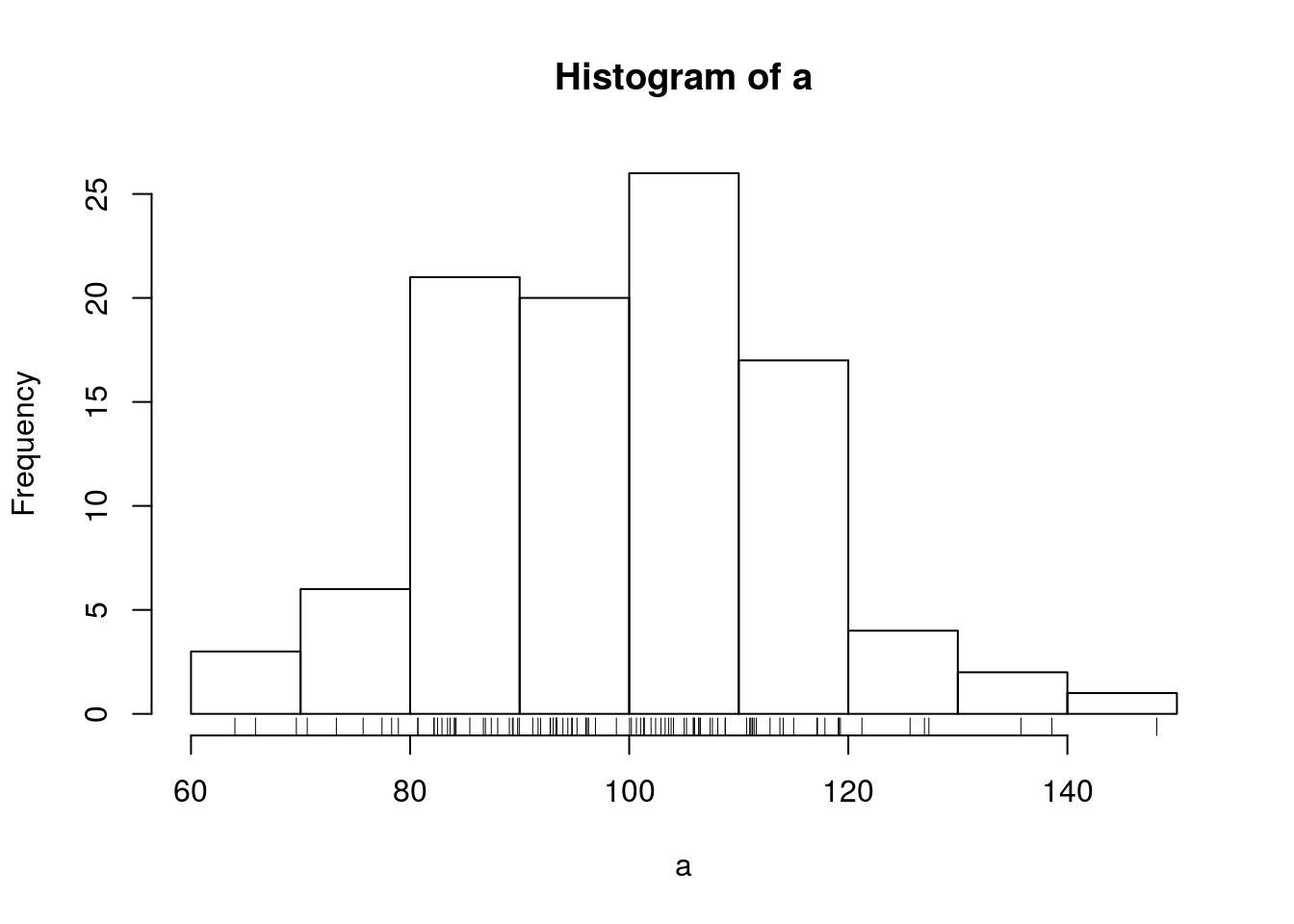plot(density(a)) abline(v=mean(a), lty=2) rug(a)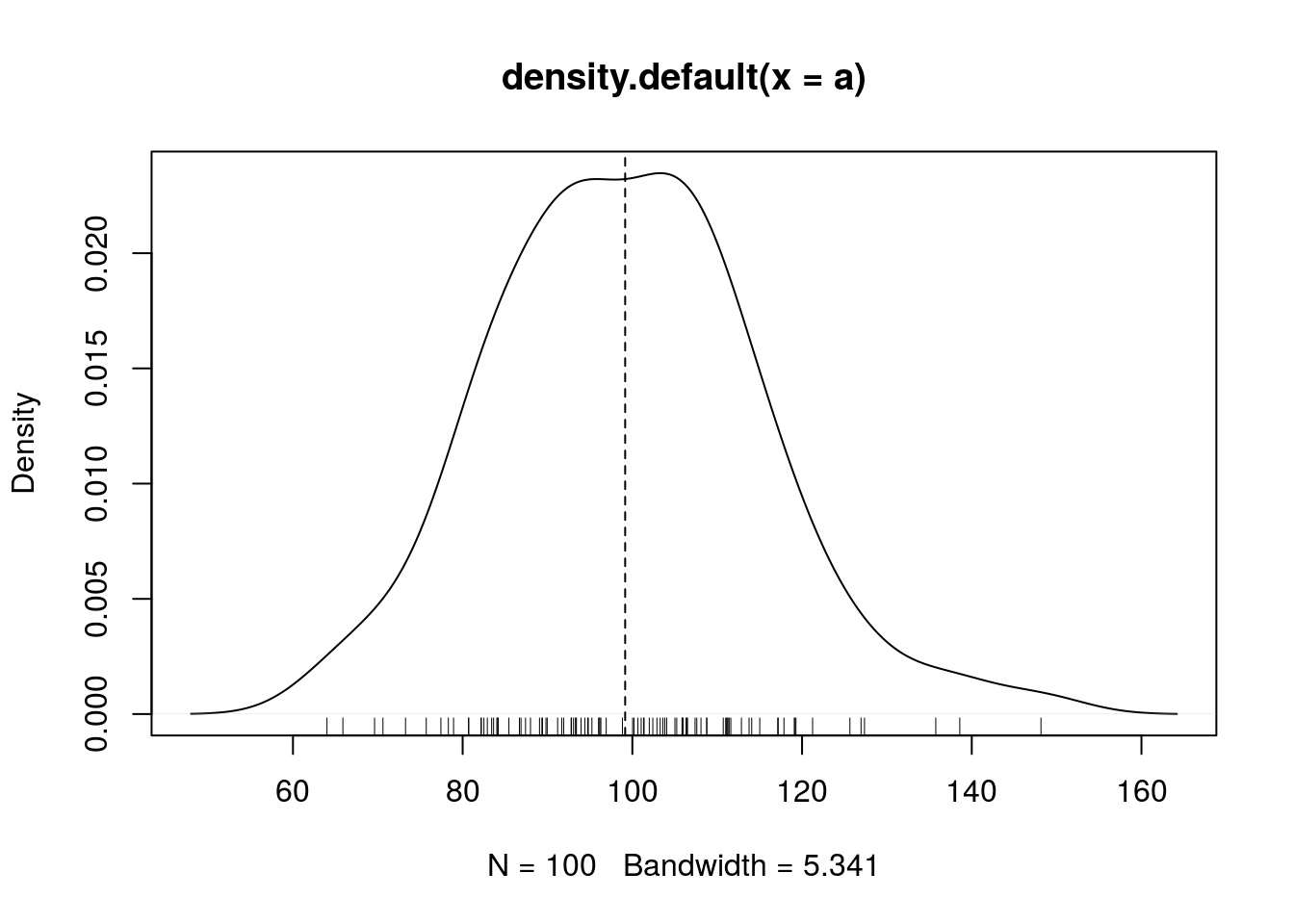boxplot(a)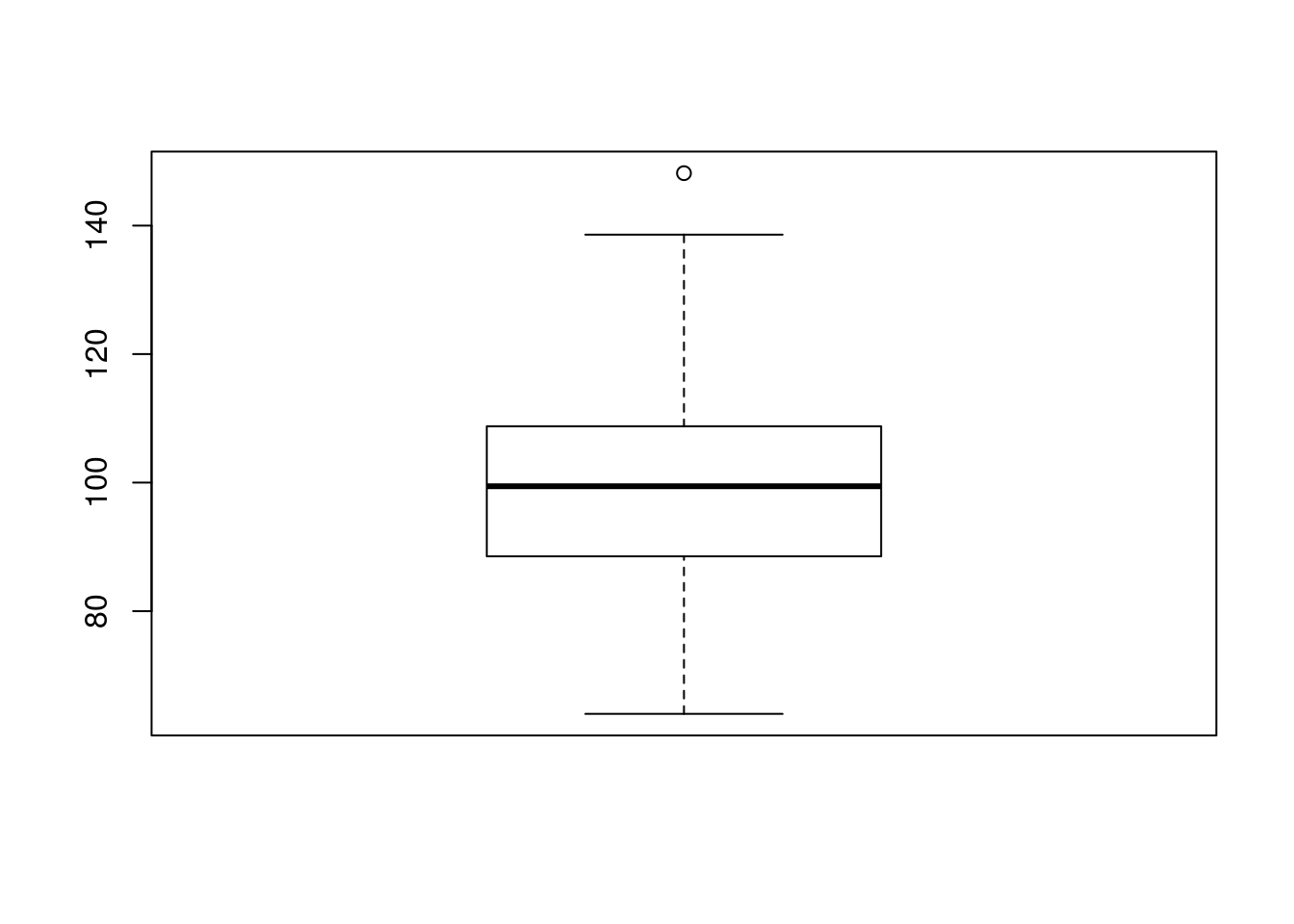## Descriptive statistics summary(a) ## Min. 1st Qu. Median Mean 3rd Qu. Max. ## 64.01 88.79 99.43 99.15 108.80 148.20 mean(a) ##  99.15297 mean(a[abs(a-mean(a)) < 2*sd(a)]) # after deleting data points beyond 2 standard deviations  ##  98.5568 ## Confidence interval of a mean Assuming normally distributed values: t.test(a) ## ## One Sample t-test ## ## data: a ## t = 63.245, df = 99, p-value < 2.2e-16 ## alternative hypothesis: true mean is not equal to 0 ## 95 percent confidence interval: ## 96.04219 102.26375 ## sample estimates: ## mean of x ## 99.15297 t.test(a)$conf.int
##   96.04219 102.26375
## attr(,"conf.level")
##  0.95

A confidence interval based on bootstrap can also be obtained:

require(boot)
## Loading required package: boot
sampmean <- function(x, d) { mean(x[d]) }
boota <- boot(a, sampmean, 1000)
boot.ci(boota)
## Warning in boot.ci(boota): bootstrap variances needed for studentized
## intervals
## BOOTSTRAP CONFIDENCE INTERVAL CALCULATIONS
## Based on 1000 bootstrap replicates
##
## CALL :
## boot.ci(boot.out = boota)
##
## Intervals :
## Level      Normal              Basic
## 95%   ( 96.14, 102.25 )   ( 96.17, 102.20 )
##
## Level     Percentile            BCa
## 95%   ( 96.10, 102.14 )   ( 96.13, 102.15 )
## Calculations and Intervals on Original Scale

# Comparing two groups (continous dependent variable)

g1 <- 500 + rnorm(30, sd=40)
g2 <- 520 + rnorm(20, sd=30)
write(g1, 'group1.dat')
write(g2, 'group2.dat')
rm(g1, g2)

Data for this example are in two text files group1.dat and group2.dat.

g1 <- scan('group1.dat')
g2 <- scan('group2.dat')

We arrange them into a data frame with two columns: group (a factor with two modalities: Gr1 and Gr2), and y which contains the values themselves.

tg <- data.frame(group=factor(rep(c('Gr1', 'Gr2'),
c(length(g1), length(g2)))),
y=c(g1, g2))

head(tg)
##   group        y
## 1   Gr1 545.8721
## 2   Gr1 543.7691
## 3   Gr1 557.8433
## 4   Gr1 483.4303
## 5   Gr1 408.2971
## 6   Gr1 537.6479
str(tg)
## 'data.frame':    50 obs. of  2 variables:
##  $group: Factor w/ 2 levels "Gr1","Gr2": 1 1 1 1 1 1 1 1 1 1 ... ##$ y    : num  546 544 558 483 408 ...
table(tg$group) ## ## Gr1 Gr2 ## 30 20 ### Graphical explorations hist(tg$y)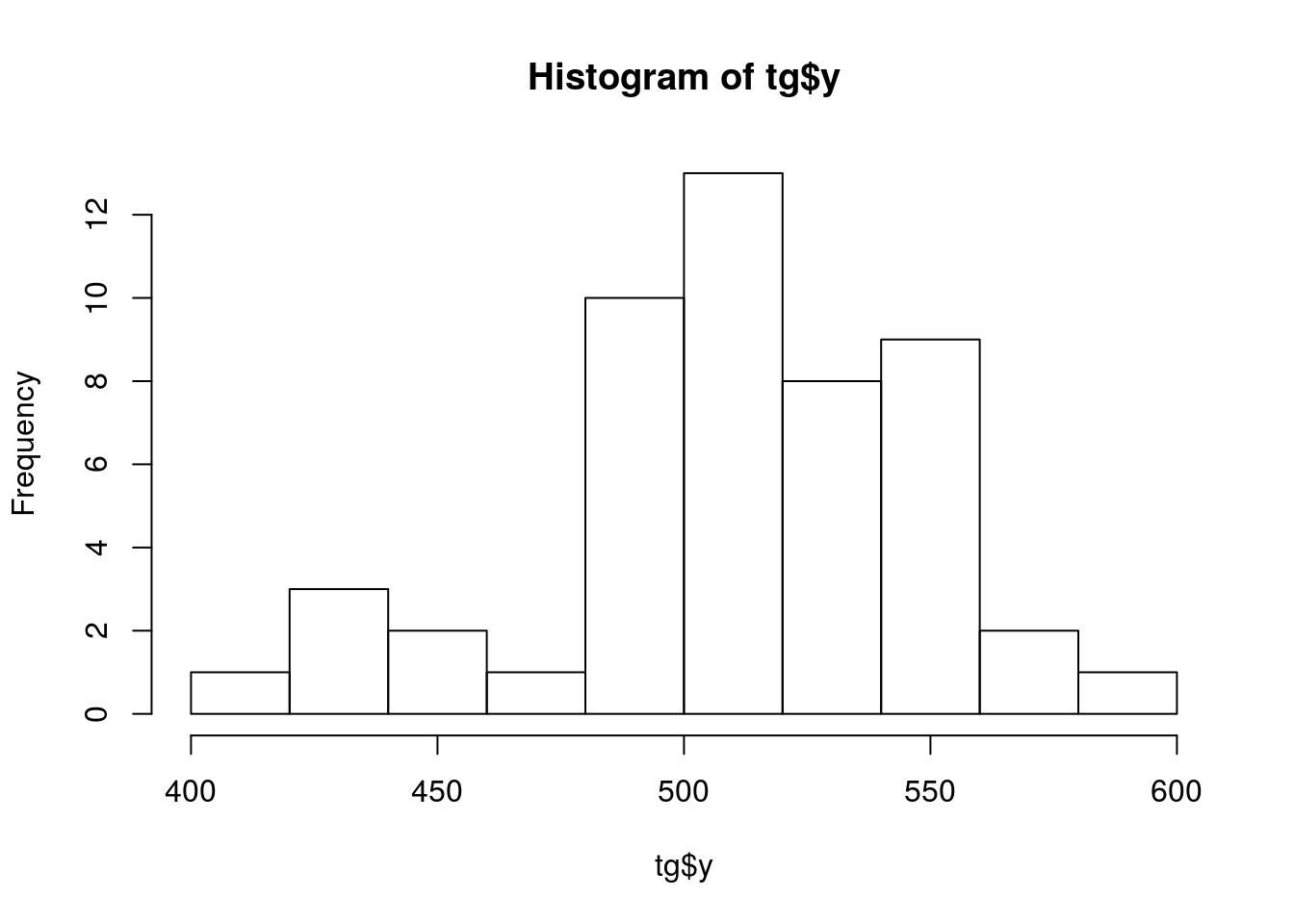boxplot(tg$y ~ tg$group)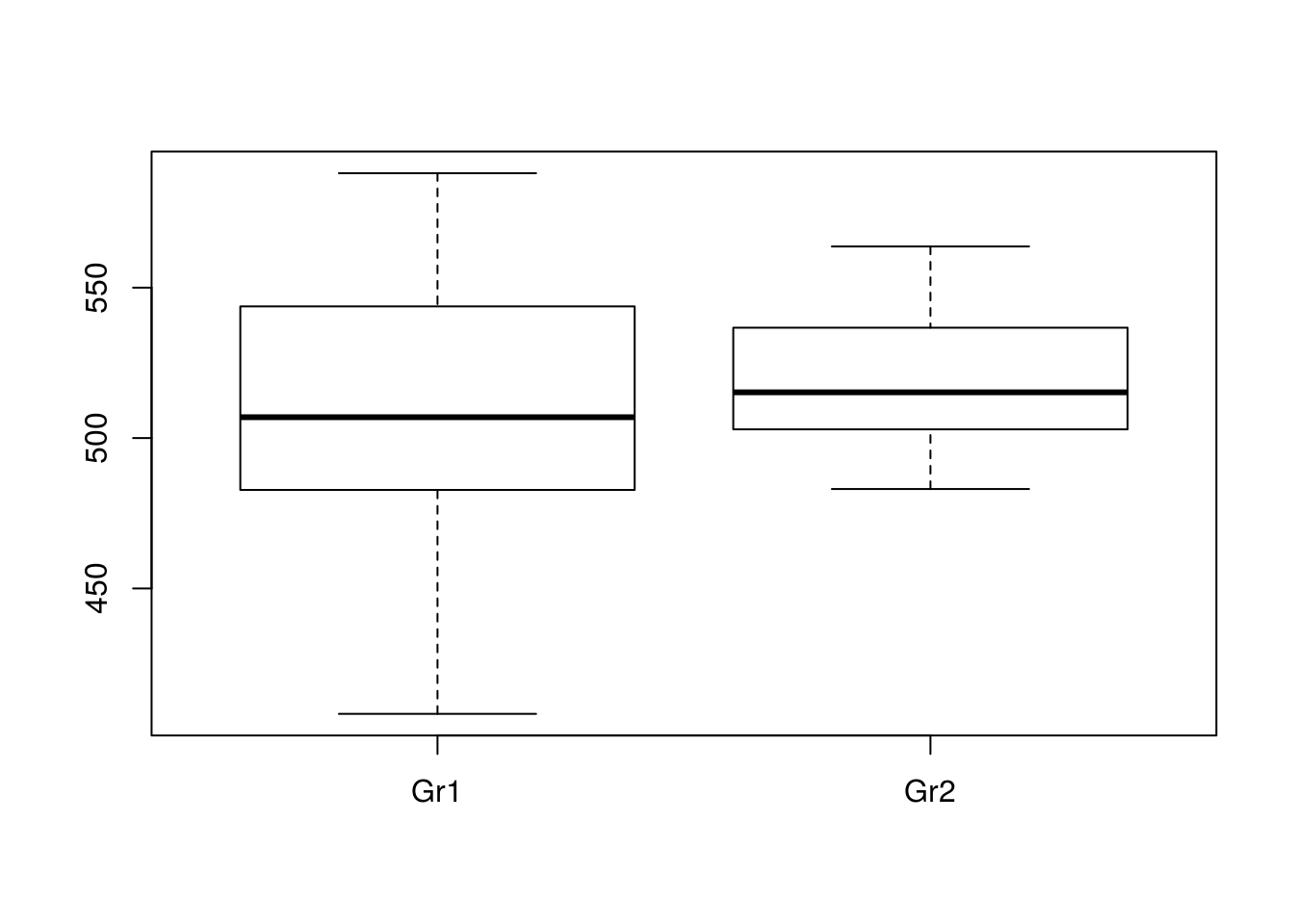require(lattice)
## Loading required package: lattice
##
## Attaching package: 'lattice'
## The following object is masked from 'package:boot':
##
##     melanoma
dotchart(tg$y, groups = tg$group)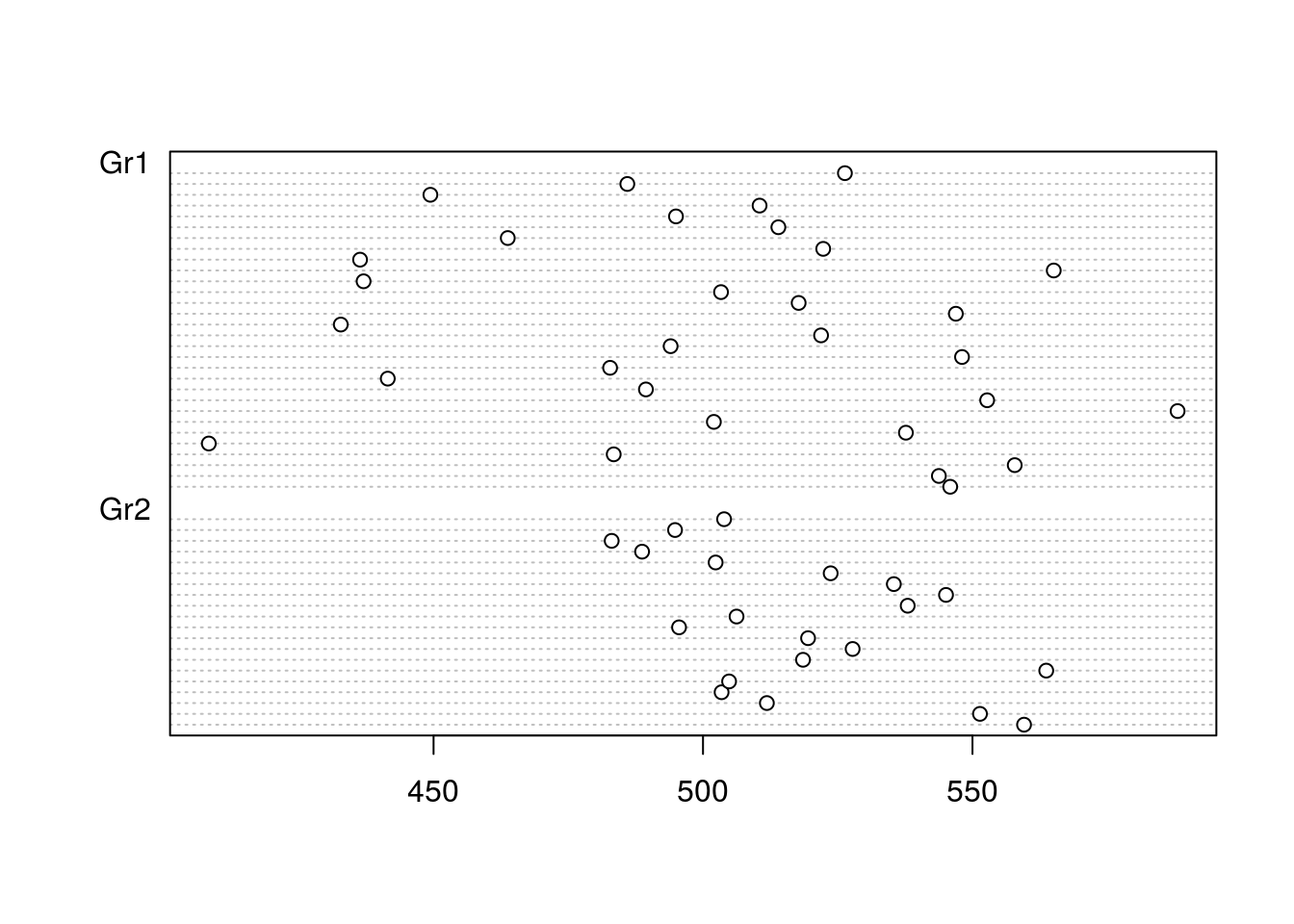When the samples are small, stripchart may be the best:

stripchart(tg$y ~ tg$group,
vertical=TRUE,
pch=1)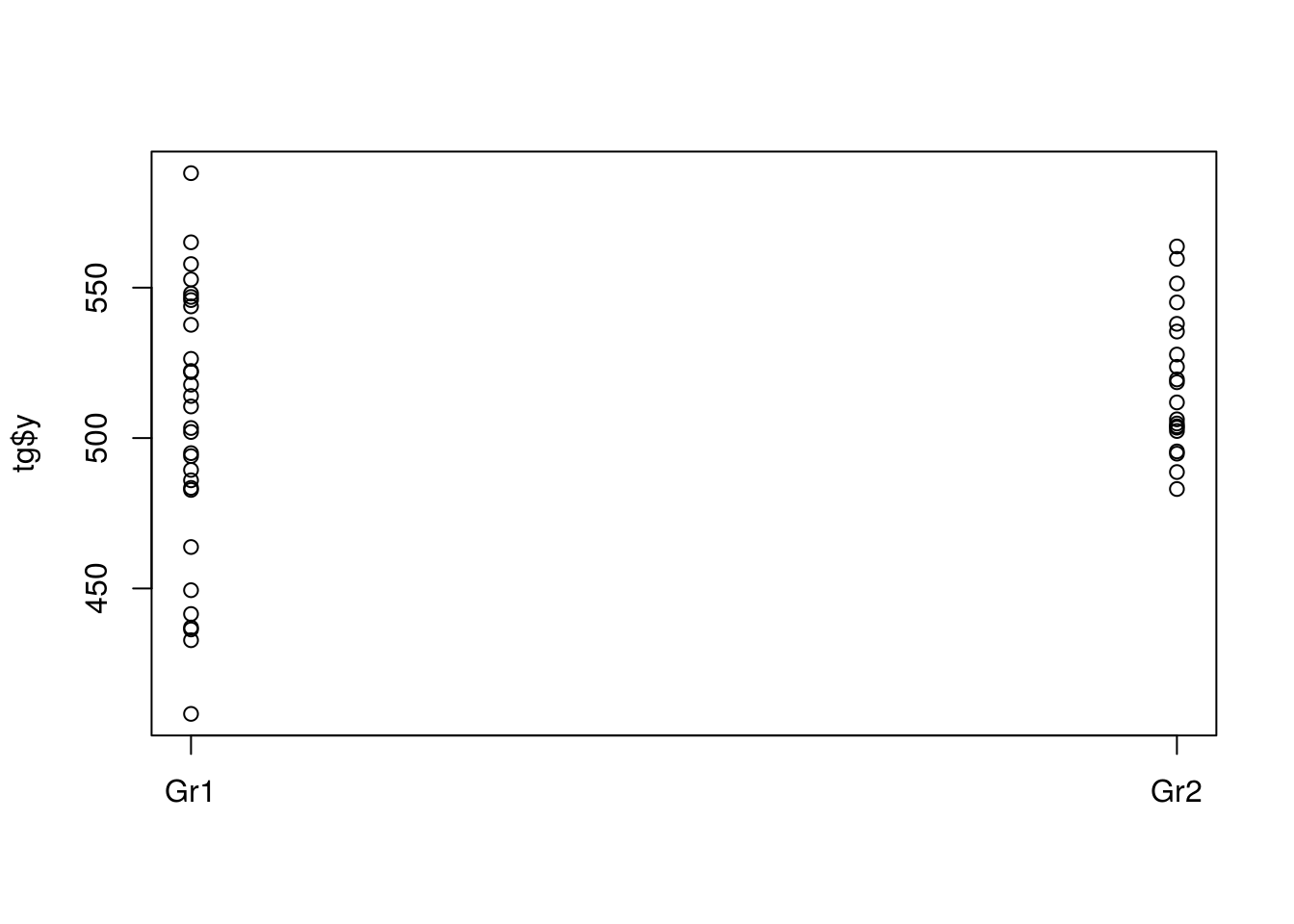If the samples are large enough, you can create density plots:

par(mfrow=(c(1, 2)))
xsca <- range(tg$y) for (gr in levels(tg$group))
{
with(subset(tg, group==gr),
{
plot(density(y), xlim=xsca, main=gr, bty='l')
rug(y, ticksize=0.1)
})
}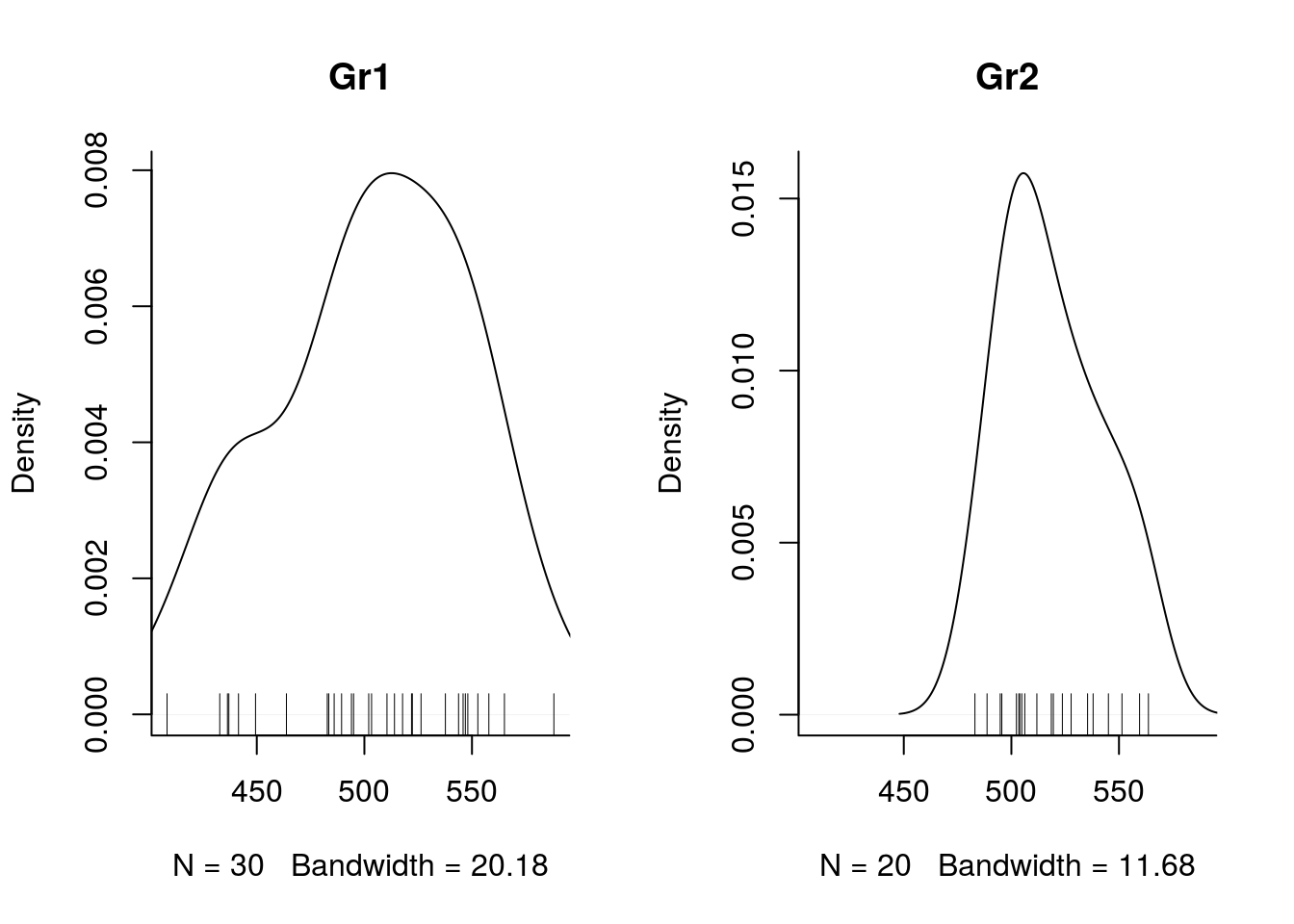Or violin plots:

require(ggplot2)
## Loading required package: ggplot2
p <-  ggplot(tg, aes(group, y))
p + geom_violin() + geom_jitter(height = 0, width = 0.1)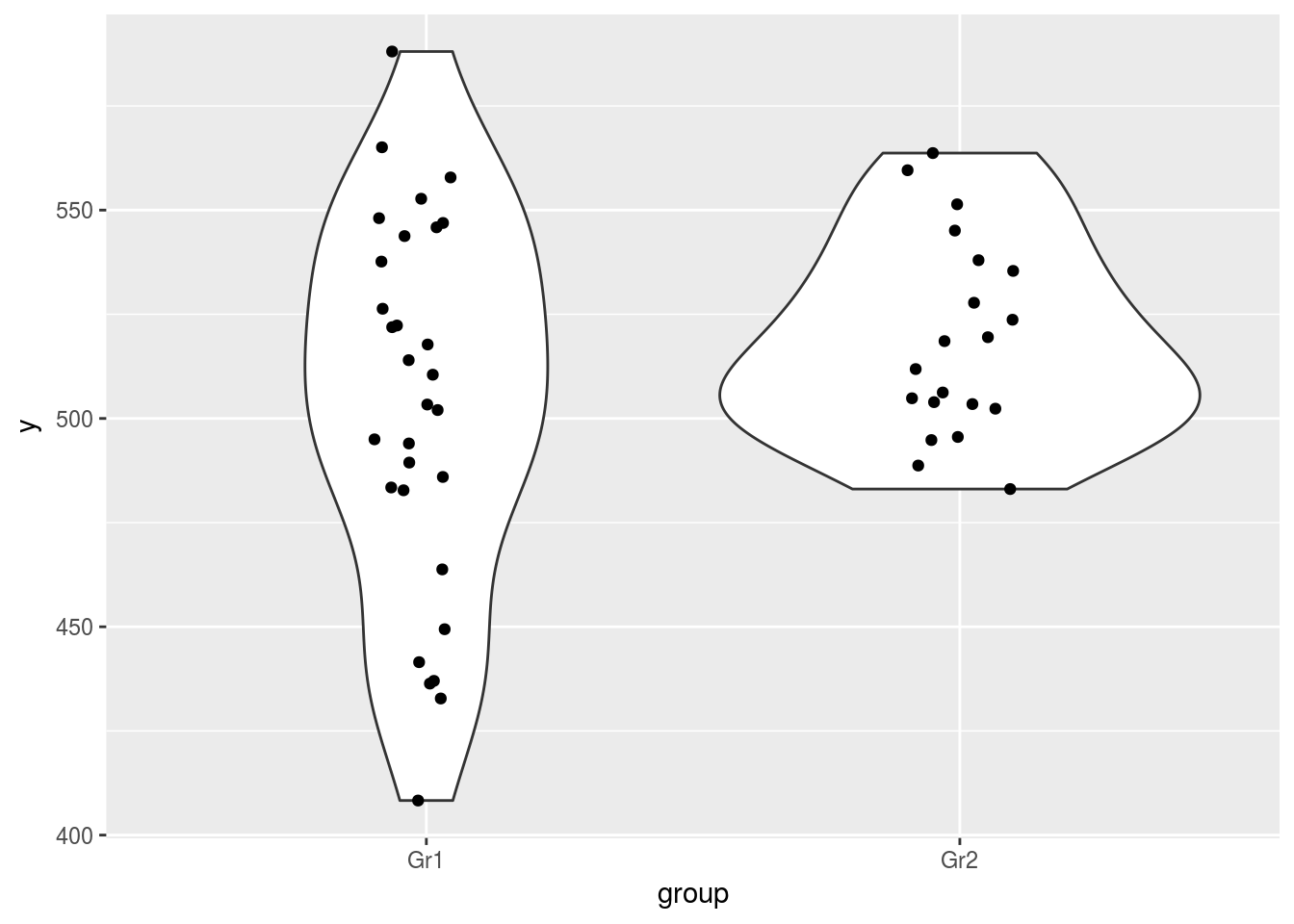To obtain the basic descriptive stats

attach(tg)
signif(tapply(y, group, mean),3)
## Gr1 Gr2
## 503 519
signif(tapply(y, group, median), 3)
## Gr1 Gr2
## 507 515
signif(tapply(y, group, sd), 3)
##  Gr1  Gr2
## 45.2 23.6
signif(tapply(y, group, se), 3)
##  Gr1  Gr2
## 8.25 5.28
detach(tg)

### Inferential statistics

Student T-tests. First assuming equal variance, then relaxing this assumption

t.test(y ~ group, data=tg, var.equal=TRUE)
##
##  Two Sample t-test
##
## data:  y by group
## t = -1.3998, df = 48, p-value = 0.168
## alternative hypothesis: true difference in means is not equal to 0
## 95 percent confidence interval:
##  -37.543155   6.724562
## sample estimates:
## mean in group Gr1 mean in group Gr2
##          503.4598          518.8691
t.test(y ~ group, data=tg)
##
##  Welch Two Sample t-test
##
## data:  y by group
## t = -1.5731, df = 45.889, p-value = 0.1226
## alternative hypothesis: true difference in means is not equal to 0
## 95 percent confidence interval:
##  -35.128392   4.309799
## sample estimates:
## mean in group Gr1 mean in group Gr2
##          503.4598          518.8691

First with a parametrisation (contr.treatment) of group where the intercept will correspond to the mean of group 1 and the effect will estimate the difference between the two groups.

contrasts(tg$group) <- contr.treatment contrasts(tg$group)
##     2
## Gr1 0
## Gr2 1
summary(lm(y ~ group, data=tg))
##
## Call:
## lm(formula = y ~ group, data = tg)
##
## Residuals:
##     Min      1Q  Median      3Q     Max
## -95.163 -19.398  -0.215  25.384  84.606
##
## Coefficients:
##             Estimate Std. Error t value Pr(>|t|)
## (Intercept)  503.460      6.962   72.31   <2e-16 ***
## group2        15.409     11.008    1.40    0.168
## ---
## Signif. codes:  0 '***' 0.001 '**' 0.01 '*' 0.05 '.' 0.1 ' ' 1
##
## Residual standard error: 38.13 on 48 degrees of freedom
## Multiple R-squared:  0.03922,    Adjusted R-squared:  0.0192
## F-statistic: 1.959 on 1 and 48 DF,  p-value: 0.168

Alternatively, one can prefer a parametrisation where the intercept estimates the global mean and the first parameter is the deviation from the global mean.

contrasts(tg$group) <- contr.sum contrasts(tg$group)
##     [,1]
## Gr1    1
## Gr2   -1
summary(lm(y ~ group, data=tg))
##
## Call:
## lm(formula = y ~ group, data = tg)
##
## Residuals:
##     Min      1Q  Median      3Q     Max
## -95.163 -19.398  -0.215  25.384  84.606
##
## Coefficients:
##             Estimate Std. Error t value Pr(>|t|)
## (Intercept)  511.164      5.504   92.87   <2e-16 ***
## group1        -7.705      5.504   -1.40    0.168
## ---
## Signif. codes:  0 '***' 0.001 '**' 0.01 '*' 0.05 '.' 0.1 ' ' 1
##
## Residual standard error: 38.13 on 48 degrees of freedom
## Multiple R-squared:  0.03922,    Adjusted R-squared:  0.0192
## F-statistic: 1.959 on 1 and 48 DF,  p-value: 0.168

### Barplot with standard errors

Barplot with the means and their associated standard errors (note this is not the standard error for the difference between the groups’ means, which is roughly $$\sqrt{2}$$ larger and, maybe for this reason, rarely used in psychology papers (like they rarely report confidence intervals))

attach(tg)
par(mfrow=c(1,1))
means <- tapply(y, group, mean)
ses <- tapply(y, group, se)

ysca = c(min(means - 3 * ses), max(means + 3 * ses))

mp <- barplot(means, ylim=ysca, xpd=F)
arrows(mp, means-ses,
mp, means+ses,
code=3, angle=90)